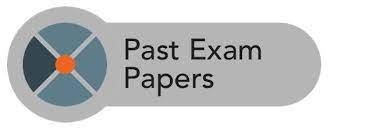Education

# JSS3 WAEC Past Questions and Answers in pdf format

JSS3 WAEC Past Questions and Answers in pdf format. Do you want to pass the JSS 3 exam? If yes, then get the jss3 past questions and answers from us. We have the complete Junior School Certificate Examination past questions and answers pdf. You can download it. You can also use the PDF version on your computer or smartphone. It will help you to pass all the subjects at one sitting.

## 👉 Relocate to Canada Today!Preparing for an examination can be a bit nerve-wracking. It gets more challenging when it is the candidate’s first time of writing an external examination. jss3 is an examination that determines the students’ opportunity to make it into Senior High School. To be admitted into the best Senior High Schools in the country requires making good grades.

On that note, let’s delve into the benefits of past questions and how they can transform your grades. These are reasons why it is important to solve past questions.

## The Benefit of Having JSS3 Past Questions

-Helps you work on your speed

Most students sometimes lose marks not necessarily because they do not have answers to the questions but because they run out of time. I know I mentioned that you can use past questions books but there is only one way you can check your speed and that is with the Past Questions.

-You can explore the answers to the questions

Questions that otherwise would have confused you in the exam hall will not get you sweating because you have the chance to find answers while solving past questions. It is important to take time to check the answers to the questions while as you solve them.

## How is Jss3 Past Questions Patterned?

We have made it simple for you. We bring all the questions which is usually in objective and theory format. We have put them together but we indicate the specific years for every question and the correct answers in order to save your time. All you need to do is to devote quality time to study the Past Questions.

## 👉 Relocate to Canada Today!

### Sample of Jss3 Past Questions and Answers on Mathematics

SECTION A: OBJECTIVE QUESTIONS:

1. What is the value of 132 – 52?
A. 12 B. 16 C. 64 D. 144 E. 194

2. Correct 0.3748 to two significant figures.
A. 0.30 B. 0.37 C. 0.38 D. 0.39 E. 0.40

3. Find the value of x, if 6/(x-1) = 2.
A. -4 B. -2 C. 4 D. 5 E. 7

4. What is the value of x in:
x + 2y = 5
x – 2y = -1?
A. 6 B. 3 C. 2 D. -2 E. -4

5. If 5/f = 7/f 4- , what is the value of f?
A. -1 B. 1/3 C. ½ D. 1 E. 3

6. The bearing of P from Q is 270o. What is the bearing of Q from P?
A. 270o B. 180o C. 150o D. 090o E. 045o.

7. Find x if, 3/(3+5) = 5/(3+x)
A. -8 B. (-16)/3 C. 16/3 D. 8 E. 17

8. Solve the following simultaneous equations.
x = y + 1
x + 2y = 7
A. (3,0) B. (3,1) C. (3,2) D. (3,3) E. (3,4)

9. One-quarter of y is equal to one and a half. What is the value of y?
A. 12 B. 10 C. 8 D. 6 E. 2

10. Simplify 3a – (9a – 5b).
A. 6a + 5b B. 6a – 5b C. -6a – 5b
D. 5b – 6a E. 12a + 5b